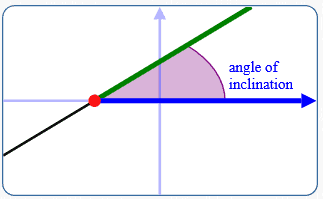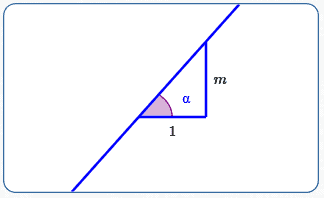﻿ Reflecting Property of a Hyperbola

# Reflecting Property of a Hyperbola

LESSON READ-THROUGH (Part 1 of 2)
by Dr. Carol JVF Burns (website creator)
Follow along with the highlighted text while you listen!
• PRACTICE (online exercises and printable worksheets)

Hyperbolas were introduced in four prior lessons:

Hyperbolas (like all conic sections) have an interesting and useful reflecting property.
In-a-nutshell: outside light aimed at one focus of the hyperbola is reflected towards the other focus.
The purpose of the current section is to explore and understand this reflecting property of a hyperbola.

## Reflecting Property of a Hyperbola

 In a hyperbola: Outside light directed towards one focus is reflected towards the other focus.You can play with this reflecting property at right: Points $F_1\,$ and $\,F_2\,$ are the foci of the hyperbola. They can be dragged to change the shape of the hyperbola. Point $\,C\,$ can also be dragged to change the shape of the hyperbola. (It controls the hyperbola constant.) Light from outside the hyperbola that is directed towards $\,F_2\,$ hits the hyperbola at point $\,\color{green}{P}\,.$ Point $\,P\,$ can be dragged around the hyperbola. The dashed grey line is the tangent to the hyperbola at point $\,P\,.$ As discussed in this earlier section, the physics Law of Reflection states that reflected light always makes equal angles with the tangent line. Observe that the reflected light always passes through $\,F_1\,$! This reflecting property has practical applications in optics and navigation.

## Why Does the Hyperbola Reflecting Property Work?

The bulk of this section is devoted to understanding why the reflecting property works.
We'll use trigonometry and also borrow a result from calculus.
The idea is simple, but (as you'll see) carrying it out requires some fortitude!

A few preliminary results are needed:

### Trigonometric Identity: Tangent of a Difference

For all real numbers $\,x\,$ and $\,y\,$ with $\,\cos(x-y)\ne 0\,,$ $$\cssId{s32}{\tan(x-y) = \frac{\tan x - \tan y}{1 + \tan x\tan y}}$$

Proof:
We want a formula that involves $\,\tan x := \frac{\sin x}{\cos x}\,$ and $\,\tan y := \frac{\sin y}{\cos y}\,.$
This fact motivates the form of ‘$\,1\,$’ that we multiply by in the re-naming below: \begin{alignat}{2} \cssId{s36}{\tan(x-y)}\ &\cssId{s37}{= \frac{\sin(x-y)}{\cos(x-y)}} &&\cssId{s38}{\text{(definition of tangent)}}\cr\cr &\cssId{s39}{= \frac{\sin x\cos y - \cos x\sin y}{\cos x\cos y + \sin x\sin y}} &\qquad&\cssId{s40}{\text{(difference formulas for sine and cosine)}}\cr\cr &\cssId{s41}{= \frac{\sin x\cos y - \cos x\sin y}{\cos x\cos y + \sin x\sin y}\cdot\frac{\frac{1}{\cos x\cos y}}{\frac{1}{\cos x\cos y}}}&&\cssId{s42}{\text{(multiply by \,1\,)}}\cr\cr &\cssId{s43}{= \frac{\frac{\sin x\color{red}{\cos y}}{\cos x\color{red}{\cos y}} - \frac{\color{red}{\cos x}\sin y}{\color{red}{\cos x}\cos y}}{\frac{\color{red}{\cos x\cos y}}{\color{red}{\cos x\cos y}} + \frac{\sin x\sin y}{\cos x\cos y}}}&&\cssId{s44}{\text{(distributive law)}}\cr\cr &\cssId{s45}{= \frac{\tan x - \tan y}{1 + \tan x\tan y}}&&\cssId{s46}{\text{(cancel, definition of tangent)}} \end{alignat} QED

### Angle of Inclination of a Line

 DEFINITION angle of inclination of a line The angle of inclination of a horizontal line equals zero. For non-horizontal lines: Every non-horizontal line in an $xy$-plane intersects the $x$-axis in a unique point. This intersection point splits both the line and the $x$-axis: the line has a part above and below the $x$-axis the $x$-axis has a part to the right and to the left of the intersection point The (nonnegative) measure of the angle between: the part of the line above the $x$-axis the part of the $x$-axis to the right of the intersection point is the angle of inclination of the line.Note:
Angle of inclination is always in the interval:
• $\,[0,180^\circ)\,$ (for degree measure)
• $\,[0,\pi)\,$ (for radian measure)

### Relationship Between Slope and Angle of Inclination of a Line

Note:
If you are visually relating slope and angle of inclination of a line,
be sure that ‘$\,1\,$’ on the $x$-axis is the same as ‘$\,1\,$’ on the $y$-axis.
Otherwise, uncomfortable things can happen!

For example, suppose ‘$\,1\,$’ on the $x$-axis is one mile to the right of zero,
but ‘$\,1\,$’ on the $y$-axis is just one inch up from zero.
Then, a line with slope $\,1\,$ appears to have an angle of inclination close to zero (instead of $\,45^\circ\,$)!

Or, suppose ‘$\,1\,$’ on the $y$-axis is one mile up from zero,
but ‘$\,1\,$’ on the $x$-axis is just one inch to the right of zero.
Then, a line with slope $\,1\,$ appears to have an angle of inclination close to $\,90^\circ\,$ (instead of $\,45^\circ\,$)!

 Fact: If $\,\alpha\,$ is the angle of inclination of any non-vertical line with slope $\,m\,,$ then: $$\cssId{s78}{\tan\alpha = m}$$ In words: the tangent of the angle of inclination of a line equals the slope of the line. Why? horizontal line: $\,\alpha = 0\,$ and $\,m = 0\,$ Thus, $\,\tan\alpha = \tan 0 = 0 = m\,.$ line with positive slope: (See top sketch at right.) Here, $\,m > 0\,.$ Thus, $\,\tan\alpha = \frac{\text{opp}}{\text{adj}} = \frac{m}{1} = m\,.$ line with negative slope: (See bottom sketch at right.) Here, $\,m < 0\,,$ so $\,-m > 0\,.$ Using the reference angle for $\,\alpha\,$ (in the green triangle), the size of $\,\tan\alpha\,$ is $\, \frac{\text{opp}}{\text{adj}} = \frac{-m}{1} = -m\,.$ Since $\,\alpha\,$ is in the second quadrant, the sign of $\,\tan\alpha\,$ is negative. Thus: $$\cssId{s97}{\tan \alpha = \overbrace{(-)}^{\text{sign}}\overbrace{(-m)}^{\text{size}} = m}$$ Note: For a vertical line, the angle of inclination is $\,\alpha = 90^\circ\,.$ In this case, both slope and $\,\tan\alpha\,$ are undefined.$$\tan(\text{angle of inclination}) = \text{slope of line}$$Finally, we need a formula for:

### Finding the (Tangent of the) Angle Between Two Intersecting Lines of Known Slope

 Suppose two non-vertical lines with known slopes intersect. As illustrated at right: Let $\,\alpha_1\,$ and $\,\alpha_2\,$ denote the angles of inclination of the two lines. Re-naming if necessary, assume $\,\alpha_2 \ge \alpha_1\,.$ Let $\,m_1\,$ be the slope of the line with angle of inclination $\,\alpha_1\,.$ Let $\,m_2\,$ be the slope of the line with angle of inclination $\,\alpha_2\,.$ Let $\,\theta\,$ be the angle between the two lines, as shown: $\,\theta := \alpha_2 - \alpha_1$ Then: \begin{alignat}{2} \cssId{s112}{\tan\theta} \ &\cssId{s113}{= \tan(\alpha_2-\alpha_1)}&\quad&\cssId{s114}{\text{(definition of \,\theta\,)}}\cr\cr &\cssId{s115}{= \frac{\tan\alpha_2 - \tan\alpha_1}{1 + \tan\alpha_2\tan\alpha_1}}&&\cssId{s116}{\text{(tangent of a difference)}}\cr\cr &\cssId{s117}{= \frac{m_2 - m_1}{1 + m_2m_1}}&&\cssId{s118}{\text{(relationship between slope and angle of inclination)}} \end{alignat}$$\tan\theta = \frac{m_2 - m_1}{1 + m_2m_1}$$ The line with the greater angle of inclination has slope $\,m_2\,.$

### Set-up/Notation for Understanding the Reflecting Property of a Hyperbola

The reflecting property of a hyperbola will be proved for a hyperbola in standard form, with foci on the $x$-axis.
As derived in Equations of Hyperbolas in Standard Form, such a hyperbola has:

• equation of the form $\,\displaystyle\frac{x^2}{a^2} - \frac{y^2}{b^2} = 1\,$ (where $\,a\,$ and $\,b\,$ are positive constants)
• the foci are $\,(c,0)\,$ and $\,(-c,0)\,,$ where $\,c\,$ is the positive number satisfying $\,c^2 = a^2 + b^2$

 Refer to the sketch at right for additional set-up: Let $\,P(x,y)\,$ be a typical point on the (right branch of) the hyperbola. The ‘outside light directed towards one focus’ is shown as the green line. The slope of the green line, from the focus $\,(c,0)\,$ to $\,P(x,y)\,,$ is: $$\cssId{sb10}{\text{slope of green line} = \frac{y_2-y_1}{x_2-x_1} = \frac{y-0}{x-c} = \frac{y}{x-c}}$$ The red line connects the other focus, $\,(-c,0)\,,$ to $\,P(x,y)\,.$ The slope of the red line is: $$\cssId{sb13}{\text{slope of red line} = \frac{y_2-y_1}{x_2-x_1} = \frac{y-0}{x-(-c)} = \frac{y}{x+c}}$$ The tangent line to the hyperbola at $\,P(x,y)\,$ is shown dashed. Borrowing a result from Calculus, the slope of this tangent line is $\displaystyle\,\frac{b^2}{a^2}\,\frac{x}{y}\,.$ For those of you who know Calculus, the details are given here. For others—preview the incredible power of Calculus! Use implicit differentiation on $\,\frac{x^2}{a^2} - \frac{y^2}{b^2} = 1\,$: $$\cssId{sb19}{\frac{2x}{a^2} - \frac{2y}{b^2}\frac{dy}{dx} = 0}$$ Solve for $\frac{dy}{dx}\,$ (the slope): $$\cssId{sb21}{\frac{dy}{dx}} \cssId{sb22}{= \frac{-2x}{a^2}\cdot\frac{b^2}{-2y}} \cssId{sb23}{= \frac{b^2}{a^2}\frac{x}{y}}$$### What Needs To Be Shown

The outside light ‘hits’ the tangent line (dashed) at $\,P(x,y)\,$ and is reflected.
By the physics Law of Reflection: $$\cssId{sb27}{\text{the red line is the path of reflected light}\qquad\text{if and only if}\qquad \alpha = \beta}$$ In other words, both of the following are true:

• If reflected light follows the red line (and thus passes through the other focus), then angle $\,\alpha\,$ equals angle $\,\beta\,.$
• If $\,\alpha = \beta\,,$ then reflected light follows the red line (and thus passes through the other focus).

Therefore:
To prove the reflecting property of the hyperbola,
we will show that $\,\alpha = \beta\,.$

Both $\,\alpha\,$ and $\,\beta\,$ are positive angles, not exceeding $\,90^\circ\,.$
The tangent function is one-to-one between $\,0^\circ\,$ and $\,90^\circ\,,$ so if $\,\tan\alpha = \tan\beta\,,$ it follows that $\,\alpha = \beta\,.$
Consequently:

To prove the reflecting property of the hyperbola,
we will show that $\,\tan\alpha = \tan\beta\,.$

Summarizing results for convenience:

• [hyperbola]
The hyperbola under investigation has equation $\displaystyle\,\frac{x^2}{a^2} - \frac{y^2}{b^2} = 1\,.$
The positive number $\,c\,$ satisfies $\,c^2 = a^2 + b^2\,.$
• [green line]
The green line is outside light directed towards the focus $\,(c,0)\,.$
This green line has slope $\displaystyle\,\frac{y}{x-c}\,,$ where $\,(x,y)\,$ are the coordinates of the point $\,P\,$ where the green line intersects the hyperbola.
• [red line]
The red line is between point $\,P(x,y)\,$ and the second focus, $\,(-c,0)\,.$
This red line has slope $\displaystyle\,\frac{y}{x+c}\,.$
• [tangent/dashed line]
The dashed line is the tangent to the hyperbola at $\,P(x,y)\,.$
It has slope $\,\displaystyle\frac{b^2}{a^2}\frac{x}{y}\,.$

Using the formula for the tangent of the angle between two intersecting lines of known slope:

• The angle $\,\alpha\,$ is formed by the intersecting green and tangent lines.
The green line has the greater angle of inclination.
Thus: $$\cssId{sb56}{\tan\alpha = \frac{\frac{y}{x-c} - \frac{b^2}{a^2}\frac{x}{y}}{1 + \frac{y}{x-c}\cdot\frac{b^2}{a^2}\cdot\frac{x}{y}}}$$
• The angle $\,\beta\,$ is formed by the intersecting red and tangent lines.
The tangent line has the greater angle of inclination.
Thus: $$\cssId{sb60}{\tan\beta = \frac{\frac{b^2}{a^2}\frac{x}{y} - \frac{y}{x+c}}{1 + \frac{b^2}{a^2}\cdot\frac{x}{y}\cdot\frac{y}{x+c}}}$$

Now—breathe deeply—and verify that the following equations are equivalent.
The final equation is true, since $\,(x,y)\,$ lies on the hyperbola $\,\displaystyle\frac{x^2}{a^2} - \frac{y^2}{b^2} = 1\,.$
Therefore, the first equation is also true, completing the proof!

\begin{alignat}{2} \tan\alpha &= \tan\beta &\qquad&\cssId{sb64}{\text{(we're trying to show this is true)}}\cr\cr \frac{\frac{y}{x-c} - \frac{b^2}{a^2}\frac{x}{y}}{1 + \frac{\color{red}{y}}{x-c}\cdot\frac{b^2}{a^2}\cdot\frac{x}{\color{red}{y}}} &= \frac{\frac{b^2}{a^2}\frac{x}{y} - \frac{y}{x+c}}{1 + \frac{b^2}{a^2}\cdot\frac{x}{\color{red}{y}}\cdot\frac{\color{red}{y}}{x+c}} &&\cssId{sb65}{\text{(substitute formulas from above)}}\cr\cr \frac{\frac{y}{x-c} - \frac{b^2}{a^2}\frac{x}{y}}{1 + \frac{x}{x-c}\cdot\frac{b^2}{a^2}} &= \frac{\frac{b^2}{a^2}\frac{x}{y} - \frac{y}{x+c}}{1 + \frac{b^2}{a^2}\cdot\frac{x}{x+c}} &&\cssId{sb66}{\text{(cancel)}}\cr\cr \frac{a^2y(x-c)}{a^2y(x-c)}\left(\frac{\frac{y}{x-c} - \frac{b^2}{a^2}\frac{x}{y}}{1 + \frac{x}{x-c}\cdot\frac{b^2}{a^2}}\right) &= \left(\frac{\frac{b^2}{a^2}\frac{x}{y} - \frac{y}{x+c}}{1 + \frac{b^2}{a^2}\cdot\frac{x}{x+c}}\right)\frac{a^2y(x+c)}{a^2y(x+c)} &&\cssId{sb67}{\text{(clear complex fractions)}}\cr\cr \frac{a^2y^2 - b^2x(x-c)}{a^2(x-c)y + b^2xy} &= \frac{b^2x(x+c) - a^2y^2}{a^2(x+c)y + b^2xy} &&\cssId{sb68}{\text{(multiply out, re-arrange factors)}}\cr\cr \bigl(a^2y^2 - b^2x(x-c)\bigr) \bigl(a^2(x+c)y + b^2xy\bigr) &= \bigl(a^2(x-c)y + b^2xy\bigr) \bigl(b^2x(x+c) - a^2y^2\bigr) &&\cssId{sb69}{\text{(cross-multiply)}}\cr\cr \bigl(a^2y^2 - b^2x^2 + b^2xc\bigr) \bigl(a^2xy + a^2cy + b^2xy\bigr) &= \bigl(a^2xy - a^2cy + b^2xy\bigr) \bigl(b^2x^2 + b^2xc - a^2y^2\bigr) &&\cssId{sb70}{\text{(distributive law)}}\cr\cr a^4xy^3 \color{purple}{+ a^4cy^3} + a^2b^2xy^3 - a^2b^2x^3y \color{red}{- a^2b^2cx^2y} - b^4x^3y &\color{red}{\,+\, a^2b^2cx^2y} + a^2b^2c^2xy \color{grey}{+ b^4cx^2y}\cr = a^2b^2x^3y \color{orange}{+ a^2b^2cx^2y} - a^4xy^3 \color{orange}{- a^2b^2cx^2y} &- a^2b^2c^2xy \color{purple}{+ a^4cy^3} + b^4x^3y \color{grey}{+ b^4cx^2y} - a^2b^2xy^3 &&\cssId{sb71}{\text{(distributive law)}}\cr\cr \color{blue}{a^4xy^3} + \color{blue}{a^2b^2xy^3} \color{green}{- a^2b^2x^3y - b^4x^3y} & + a^2b^2c^2xy\cr = \color{green}{a^2b^2x^3y} \color{blue}{- a^4xy^3} &- a^2b^2c^2xy \color{green}{+ b^4x^3y} \color{blue}{- a^2b^2xy^3} &&\cssId{sb72}{\text{(delete red/orange/purple/grey terms)}}\cr\cr \color{blue}{xy^3(2a^4 + 2a^2b^2)} + \color{green}{x^3y(-2a^2b^2 - 2b^4)} &+ xy(2a^2b^2c^2) = 0 &&\cssId{sb73}{\text{(gather like terms on left side)}}\cr\cr y^2(a^4 + a^2b^2) + x^2(-a^2b^2 - b^4) &+ a^2b^2c^2 = 0 &&\cssId{sb74}{\text{(divide by \,2xy\,)}}\cr\cr y^2a^2(a^2 + b^2) - x^2b^2(a^2 + b^2) &+ a^2b^2c^2 = 0 &&\cssId{sb75}{\text{(factor)}}\cr\cr y^2a^2c^2 - x^2b^2c^2 &+ a^2b^2c^2 = 0 &&\cssId{sb76}{\text{(\,a^2 + b^2 = c^2\,)}}\cr\cr - y^2a^2 + x^2b^2 &- a^2b^2 = 0 &&\cssId{sb77}{\text{(multiply by \,-1\,, divide by \,c^2\,)}}\cr\cr x^2b^2 - y^2a^2 &= a^2b^2 &&\cssId{sb78}{\text{(re-arrange)}}\cr\cr \frac{x^2}{a^2} - \frac{y^2}{b^2} &= 1 &&\cssId{sb79}{\text{(divide by \,a^2b^2\,)}}\cr\cr \end{alignat}

Hooray!
Isn't it wonderful when simplicity emerges from seeming chaos?

Master the ideas from this section